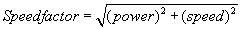# Title 40

## SECTION 94.107

### 94.107 Determination of maximum test speed.

§ 94.107 Determination of maximum test speed.

(a) Overview. This section specifies how to determine maximum test speed from a lug curve. This maximum test speed is used in §§ 94.105, 94.106, and § 94.109 (including the tolerances for engine speed specified in § 94.105).

(b) Generation of lug curve. Prior to beginning emission testing, generate maximum measured brakepower versus engine speed data points using the applicable method specified in 40 CFR 1065.510. These data points form the lug curve. It is not necessary to generate the entire lug curve. For the portion of the curve where power increases with increasing speed, it is not necessary to generate points with power less than 90 percent of the maximum power value. For the portion of the curve where power decreases with increasing speed, it is not necessary to generate points with power less than 75 percent of the maximum power value.

(c) Normalization of lug curve. (1) Identify the point (power and speed) on the lug curve at which maximum power occurs.

(2) Normalize the power values of the lug curve by dividing them by the maximum power value identified in paragraph (b)(1) of this section, and multiplying the resulting values by 100.

(3) Normalize the engine speed values of the lug curve by dividing them by the speed at which maximum power occurs, which is identified in paragraph (b)(1) of this section, and multiplying the resulting values by 100.

(4) Maximum engine power is located on the normalized lug curve at 100 percent power and 100 percent speed.

(d) Determination of maximum test speed. Calculate the maximum test speed from the speedfactor analysis described in this paragraph (d).

(1) For a given combination of engine power and speed (i.e., a given power/speed point), the speedfactor is the distance to the normalized power/speed point from the zero power, zero speed point. The value of the speedfactor is defined as:(2) Calculate speedfactors for the power/speed data points on the lug curve, and determine the maximum value.

(3) Maximum test speed is the speed at which the maximum value for the speedfactor occurs.

(e) For constant-speed engines, rated speed is the maximum test speed.

(f) For Category 3 engines, manufacturers may choose to set the maximum test speed at the maximum in-use engine speed instead of the speed specified in § 94.107(d).

[64 FR 73331, Dec. 29, 1999, as amended at 68 FR 9784, Feb. 28, 2003; 70 FR 40458, July 13, 2005]